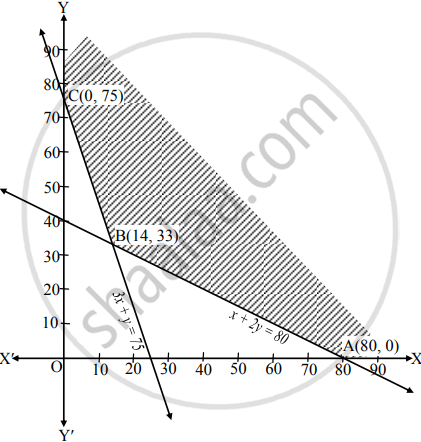# Solve the following problem : A factory produced two types of chemicals A and B The following table gives the units of ingredients P and Q (per kg) of Chemicals A and B as well as minimum requirement - Mathematics and Statistics

Graph
Sum

Solve the following problem :

A factory produced two types of chemicals A and B The following table gives the units of ingredients P & Q (per kg) of Chemicals A and B as well as minimum requirements of P and Q and also cost per kg. of chemicals A and B.

 Ingredients per kg. /Chemical Units A (x) B (y) Minimum requirements in P 1 2 80 Q 3 1 75 Cost (in ₹) 4 6

Find the number of units of chemicals A and B should be produced so as to minimize the cost.

#### Solution

Let the factory produces ‘x’ units of chemical A and ‘y’ units of chemical B∴ Total cost Z = 4x + 6y
This is the objective function to be minimized.
From the given information, the constraints are
x + 2y ≥ 80, 3x + y ≥ 75, x ≥ 0, y ≥ 0
∴ Given problem can be formulated as
Minimize Z = 4x + 6y
Subject to, x + 2y ≥ 80, 3x + y ≥ 75, x ≥ 0, y ≥ 0
To draw the feasible region, construct table as follows:

 Inequality x + 2y ≥ 80 3x + y ≥ 75 Corresponding equation (of line) x + 2y = 80 3x + y = 75 Intersection of line with X-axis (80, 0) (25, 0) Intersection of line with Y-axis (0, 40) (0, 75) Region Non-origin side Non-origin side

Shaded portion XABCY is the feasible region,
whose vertices are A ≡ (80, 0), B and C ≡ (0, 75)
B is the point of intersection of the lines 3x + y = 75 and x + 2y = 80
Solving the above equations, we get
B ≡ (14, 33)
Here the objective function is,
Z = 4x + 6y
∴ Z at A(80, 0) = 4(80) + 6(0) = 320
Z at B(14, 33) = 4(14) + 6(33) = 254
Z at C(0, 75) = 4(0) + 6(75) = 450
∴ Z has minimum value 254 at B(14, 33)
∴ Z is mimimum, when x = 14, y = 33.
∴ Factory should produce 14 units of chemical A and 33 units of chemical B to minimize the cost to ₹ 254.

Concept: Mathematical Formulation of Linear Programming Problem
Is there an error in this question or solution?

#### APPEARS IN

Balbharati Mathematics and Statistics 2 (Commerce) 12th Standard HSC Maharashtra State Board
Chapter 6 Linear Programming
Miscellaneous Exercise 6 | Q 4.1 | Page 104